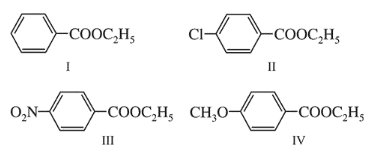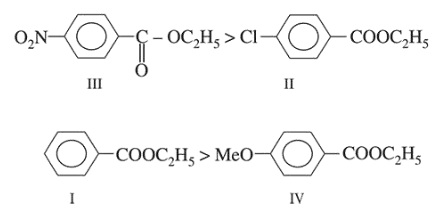# The decreasing order of ease of alkaline hydrolysis for the following esters isQuestion:

The decreasing order of ease of alkaline hydrolysis for the following esters is1. III > II > IV > I

2. $\mathrm{III}>\mathrm{II}>\mathrm{I}>\mathrm{IV}$

3. $\mathrm{IV}>\mathrm{II}>\mathrm{III}>\mathrm{I}$

4. $\mathrm{II}>\mathrm{III}>\mathrm{I}>\mathrm{IV}$

Correct Option: , 2

Solution:

Rate of reaction $\propto$ Electrophilicity of carbonyl carbon, so, E.W.G. increases rate while E.R.G. decreases the rate.